## 9.5. Limit Cycles and Chaos

We found that Ricker's model is stable if 0 < r < 2. The question is what happens to model population when stability is lost. The first suggestion is that population will get extinct. But this is the wrong answer. Below there are simulations of population dynamics using Ricker's model with different values of r: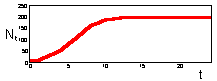r = 0.5 Monotonous increase in numbers.K = 200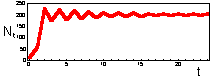r = 1.9 Damping oscillations.K = 200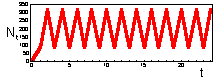r = 2.3 Limit cycle with period = 2.K = 200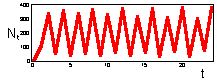r = 2.6 Limit cycle with period = 4.K = 200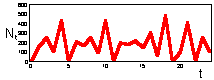r = 3.0 Chaos.K = 200
In the two upper figures the model has a stable equilibrium, only the patterns of approaching the equilibrium are different. In the three lower figures there is no stable equilibrium. Non-equilibrium dynamics may be of 2 types: a limit cycle when the trajectory repeats itself, and chaotic when the trajectory does not repeat itself.

The bifurcation plot (below) helps to visualize all types of dynamics generated by the Ricker's model. This graph is plotted as follows: for each value of parameter r which is incremented with 0.05 steps, population dynamics was simulated for 200 generations. First 125 generations were discarded because the population may not have reached the asymptotic behavior. Population numbers in the rest 75 generations are plotted versus the value of parameter r:• r < 2 Stable equilibrium
• r = 2 Bifurcation into a 2-point limit cycle
• r = 2.5 Bifurcation into a 4-point limit cycle
• Then there is a series of cycle duplication: 8 points, 16 points, etc.
• r = 2.692 Chaos ("smear" of points)
• For r > 2.7 there are some regions where dynamics returns to a limit cycle, e.g., r=3.15.
Chaotic dynamics looks like stochastic noise, however the model is absolutely deterministic. Chaotic models are widely used for random number generation in computers.

One of the questions that are often discussed in ecological literature is "does chaos really exists in population dynamics?". The major argument in favor of chaos is: when model parameters are fit to known time series of population dynamics then the dynamics of the model with these parameters is chaotic. Another kind of arguments is based on attempts to separate chaotic dynamics from stochastic noise. However detection of chaos is difficult because of several problems:

• There is no evidence that the model is correct. Usually these models ignore many ecological processes (natural enemies, etc.). It is necessary to use multiple models for detecting chaos (Ellner and Turchin 1995, Amer. Natur. 145: 343-375).
• The confidence interval for parameter values is usually large enough to cover both chaotic and non-chaotic (limit cycle) model dynamics.
• Time series of population dynamics are usually not long enough to separate chaotic dynamics from stochastic noise.
At this point, chaotic dynamics was detected consistently only in a few microtine populations (Ellner and Turchin 1995). I suspect that in these cases chaotic dynamics was induced by the seasonal cycle in population numbers. Chaos was never detected in time series with 1 year as a time step. Probably chaos is a rare phenomenon in population dynamics.

Alexei Sharov 1/12/96